# Quant Quiz for IBPS CLERK MAINS 2018 | 8th January 2019

Quant Quiz to improve your Quantitative Aptitude & Data Interpretation. For upcoming exams like Bank, SSC, Railway, UPSC, UPSSSC, CDS, UPTET, KVS, DSSSB and other Government exams.

Directions (Q1-Q5): Percentage distribution of Income of seven firms in year 2015 and 2016 is given below in pie charts. Percentage distribution of some firms are not given in the chart. You have to calculate these values if required to answer the question.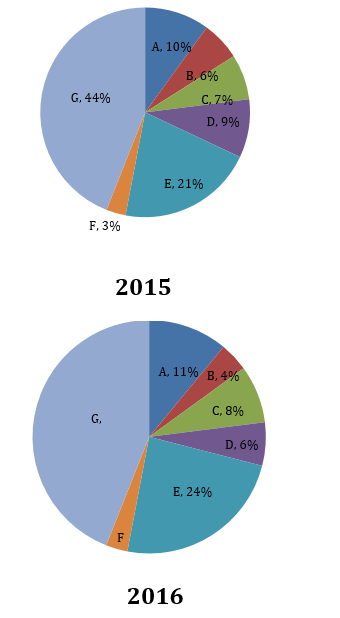Note Ratio of Total Income of all 7 firms in 2015 to total income of all seven firms in 2016 5 : 7.

Q1. If profit percent earned by company C in 2015 and profit percent earned by company       D in 2016 are equal and income of company D in 2016 is 10 million and expenditure of company D in 2016 is 8 million then what will be profit of C in 2015?Q2. If total income of all firm in 2015 is 13860 million then what is the difference between the income of firm E in 2015 and income of A in 2016.

(a) 776 m

(b) 820 m

(c) 720 m

(d) 810 m

(e) None of these

Q3. What will be the ratio of income of firm D in 2015 to the income of firm G in 2016 if income of G and F in 2016 is in the ratio of 24 : 23?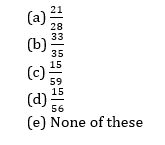Q4. If difference between the total income of all firms in 2015 and 2016 in N then what will be the difference between the average of income of firm A, B and C together in 2015 and average of income of firm B, C and D together in 2016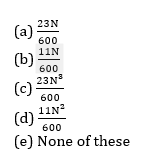Q5. If income of company G in 2016 is % more than income of company G in 2015 then what is the percentage distribution of income for firm F in 2016.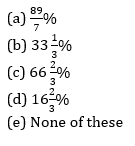Directions (Q6–Q10): What will come in place of the question-mark (?) in the following questions:

Q6. 16.5% of 1399.921 + 114.78% of 1211 = ?

(a) 1270

(b) 1350

(c) 1490

(d) 1530

(e) 1610

Q7.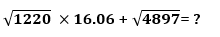(a) 610

(b) 620

(c) 630

(d) 640

(e) 650

Q8.  18.08 11.898 + 22.922  14.94 = ?

(a) 520

(b) 560

(c) 540

(d) 580

(e) 610

Q9.(a) 280.4

(b) 290.4

(c) 295.4

(d) 285.4

(e) None of these

Q10.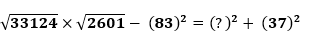(a) 37

(b) 33

(c) 34

(d) 28

(e) None of these

# Solutions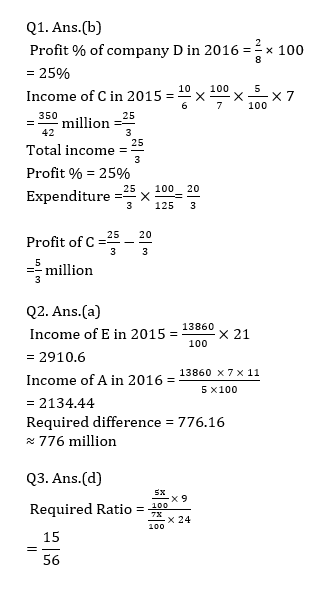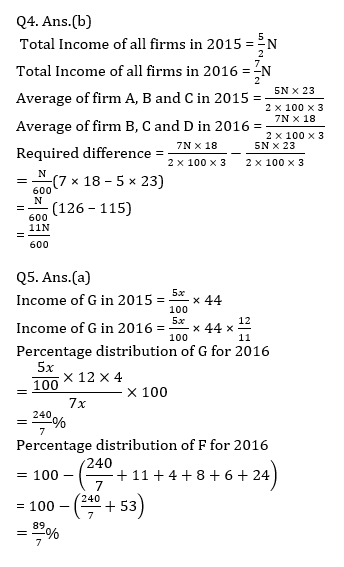## WhatsApp Group Join here

Mail us at : ambitiousbaba1@gmail.com